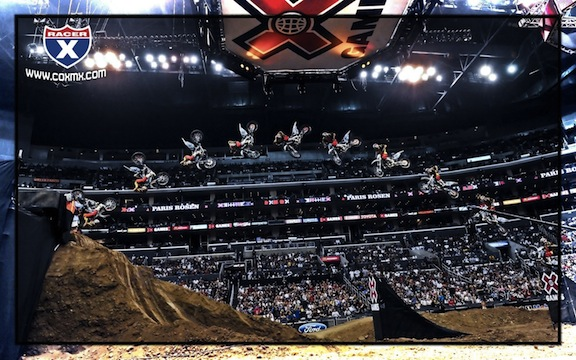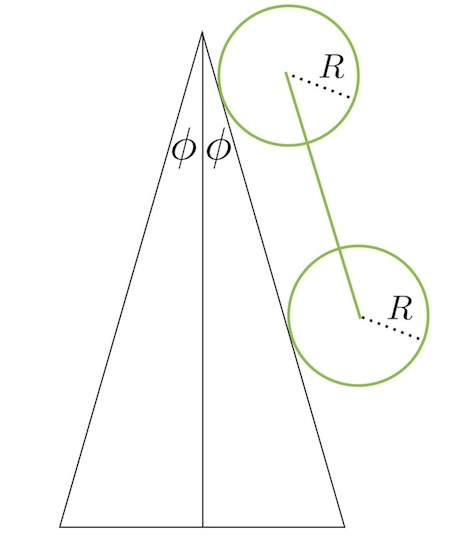Motocross Front FlipIn 2011, Jackson Strong won the gold medal for freestyle motocross best trick at the summer X Games for a front flip over a sixty foot gap, the first in competition. Powering a dirt bike through a front flip is no easy task and requires an incredible combination of balance, strategic shifting of body weight, and... pulling the brakes. Let's explore the role of the brakes in facilitating the front flip.

We model model a dirt bike as a rod of mass $M = \SI{100}{\kilo\gram},$ and length $L = \SI{2}{\meter}$ to which are attached two wheels of mass $m =\SI{40}{\kilo\gram},$ and radius $R = \SI{0.5}{\meter}.$ The bike goes off a spine ramp with angle $\displaystyle \phi=1^{\circ}$ to the vertical on either side (see diagram below).

Our question is, at what time, $t_\textrm{pull}$ (in seconds), after leaving the ramp, should the rider pull the brakes (stopping both wheels instantaneously) if they want to do a front flip with minimum takeoff speed?Details and assumptions

• Upon landing, the bike is parallel to the landing ramp, both wheels are in contact with the landing surface, and the back wheel contacts the very top of the ramp.
• The wheels are rings, i.e. the spokes are massless and all the mass is concentrated at the radius and $I_\textrm{wheel} = mR^2$.
• Assume both wheels lose contact with the ramp at the same angular velocity, $v_0/R$, where $v_0$ is the velocity of the motorcycle upon losing contact with the ramp.
• The bike coasts off the ramp, i.e. there is no power from the engine putting a torque on the wheel.
×